# hitprob

Compute Markov chain hitting probabilities

Since R2019b

## Syntax

``hp = hitprob(mc,target)``
``hp = hitprob(mc,target,'Graph',true)``
``[hp,h] = hitprob(mc,target,'Graph',true)``
``[hp,h] = hitprob(ax,mc,target,'Graph',true)``

## Description

example

``hp = hitprob(mc,target)` returns the probability `hp` of hitting a specified subset of states `target`, beginning from each state in the Markov chain `mc`. If `target` forms a recurrent class, the elements of `hp` are absorption probabilities.`

example

``hp = hitprob(mc,target,'Graph',true)` plots a directed graph of `mc` with node colors representing the hitting probabilities. A color bar summarizes the color coding.`
````[hp,h] = hitprob(mc,target,'Graph',true)` also returns the plot handle. Use `h` to modify properties of the plot after you create it.```
``` `[hp,h] = hitprob(ax,mc,target,'Graph',true)` plots on the axes specified by `ax` instead of the current axes (`gca`).```

## Examples

collapse all

Consider this theoretical, right-stochastic transition matrix of a stochastic process.

`$P=\left[\begin{array}{cccc}1& 0& 0& 0\\ 1/2& 0& 1/2& 0\\ 0& 1/2& 0& 1/2\\ 0& 0& 0& 1\end{array}\right].$`

Create the Markov chain that is characterized by the transition matrix P.

```P = [1 0 0 0; 1/2 0 1/2 0; 0 1/2 0 1/2; 0 0 0 1]; mc = dtmc(P);```

Plot a directed graph of the Markov chain. Visually identify the communicating class to which each state belongs by using node colors.

```figure; graphplot(mc,'ColorNodes',true);```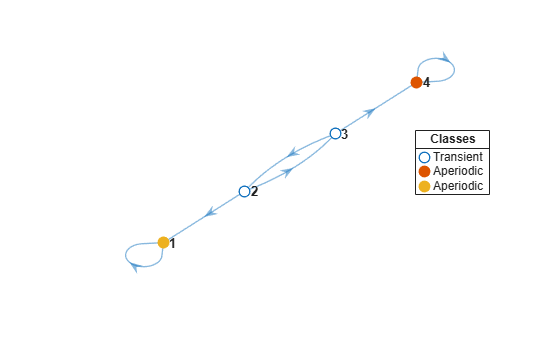Compute the hitting probabilities for state 1, beginning from each state in the Markov chain.

`hp = hitprob(mc,1)`
```hp = 4×1 1.0000 0.6667 0.3333 0 ```

Because state 1 is the target, the probability of state 1 reaching itself is `1`.

State 1 is reachable from states 2 and 3. Therefore, the hitting probabilities for state 1 beginning from those states are positive.

Because state 1 is unreachable from state 4, state 4 has a hitting probability of `0` for state 1. Therefore, state 4 is a remote state with respect to state 1.

Consider this theoretical, right-stochastic transition matrix of a stochastic process.

`$P=\left[\begin{array}{ccccccc}1/2& 0& 1/2& 0& 0& 0& 0\\ 0& 1/3& 0& 2/3& 0& 0& 0\\ 1/4& 0& 3/4& 0& 0& 0& 0\\ 0& 2/3& 0& 1/3& 0& 0& 0\\ 1/4& 1/8& 1/8& 1/8& 1/4& 1/8& 0\\ 1/2& 0& 0& 0& 0& 0& 1/2\end{array}\right].$`

Create the Markov chain that is characterized by the transition matrix P.

```P = [1/2 0 1/2 0 0 0 0 0 1/3 0 2/3 0 0 0 1/4 0 3/4 0 0 0 0 0 2/3 0 1/3 0 0 0 1/4 1/8 1/8 1/8 1/4 1/8 0 1/6 1/6 1/6 1/6 1/6 1/6 0 1/2 0 0 0 0 0 1/2]; mc = dtmc(P);```

Plot a digraph of the Markov chain `mc`. Specify node colors representing the hitting probabilities for state 1, beginning from each state in the Markov chain.

`hitprob(mc,1,'Graph',true);`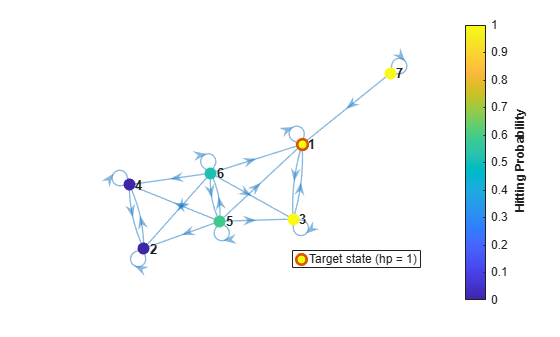Plot another digraph. Include state 3 as a target state.

`hitprob(mc,[1 3],'Graph',true);`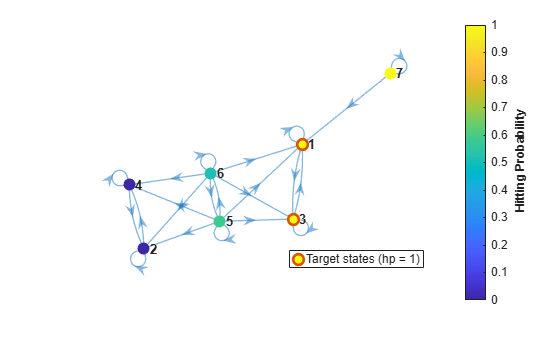The probability of hitting states 1 or 3 from state 6 is approximately 0.5.

Create a 20-state Markov chain from a random transition matrix containing 375 randomly placed infeasible transitions. An infeasible transition is a transition whose probability of occurring is zero.

```rng(4) % For reproducibility mc = mcmix(20,'Zeros',375);```

Plot a digraph showing, for each state, the probability of transitioning to the subclass containing states 1 and 2.

```target = [1 2]; hitprob(mc,target,'Graph',true);```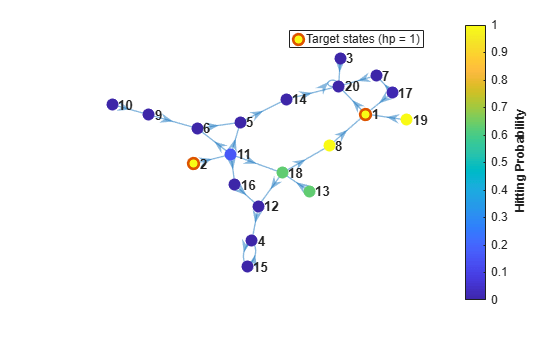Create a 20-state Markov chain from a random transition matrix containing 375 randomly placed infeasible transitions. Plot a digraph of the Markov chain.

```rng(4) mc = mcmix(20,'Zeros',375);```

Find a recurrent class in the Markov chain `mc` by following this procedure:

1. Classify the states by passing `mc` to `classify`. Return the array of class memberships `ClassStates` and the logical vector specifying whether the classes are recurrent `ClassRecurrence`.

2. Extract the recurrent classes from the array of classes by indexing into the array using the logical vector.

```[~,ClassStates,ClassRecurrence] = classify(mc); s = ClassStates{ClassRecurrence}```
```s = 1x2 string "4" "15" ```

States 4 and 15 form a recurrent class.

Plot two digraphs of the Markov chain `mc`. For the first digraph, use node colors to identify the state classification. For the second digraph, show the probability of absorption into the recurrent class for each state.

```subplot(2,1,1) graphplot(mc,'ColorNodes',true) legend off subplot(2,1,2) hitprob(mc,s,'Graph',true);```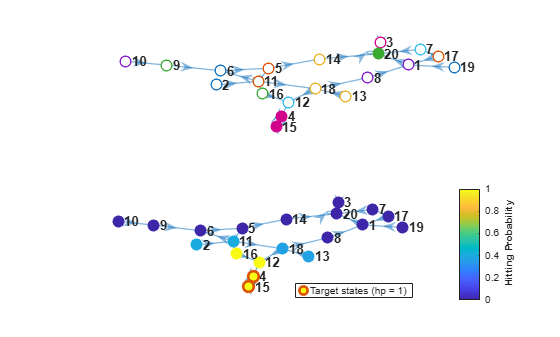The states to the left of states 2, 11, 18, and 13 are remote with respect to the recurrent class. Therefore, their absorption probability is 0.

## Input Arguments

collapse all

Discrete-time Markov chain with `NumStates` states and transition matrix `P`, specified as a `dtmc` object. `P` must be fully specified (no `NaN` entries).

Target subset of states, specified as a numeric vector of positive integers, string vector, or cell vector of character vectors.

• For a numeric vector, elements of `target` correspond to rows of the transition matrix `mc.P`.

• For a string vector or cell vector of character vectors, elements of `target` must be state names in `mc.StateNames`.

Example: `["Regime 1" "Regime 2"]`

Data Types: `double` | `string` | `cell`

Axes on which to plot, specified as an `Axes` object.

By default, `hitprob` plots to the current axes (`gca`).

## Output Arguments

collapse all

Hitting probabilities, returned as a numeric vector of length `mc.NumStates`. `hp(i)` is the probability of hitting the specified subset of the target states `target` from starting state `i`.

`hp` is not a probability distribution; its elements do not have to sum to `1`.

Handle to the graph plot, returned as a graphics object when the `'Graph'` name-value pair argument is `true`. `h` is a unique identifier, which you can use to query or modify properties of the plot.

collapse all

### Remote State

Remote states are those states from which the target states are unreachable. A remote state has a hitting probability of `0` and an expected first hitting time of `Inf`. For more details on expected first hitting times, see `hittime`.

## Algorithms

`hitprob` uses `linprog` to find the minimum norm nonnegative solution to the system:

`$\left\{\begin{array}{cc}{h}_{i}^{A}=1& ,i\in A\\ {h}_{i}^{A}=\sum _{j=1}^{N}{P}_{ij}{h}_{j}^{A}& ,i\notin A,\end{array}$`

where

• ${h}_{i}^{A}$ = `hp(i)`, the probability of hitting the subset of states A, beginning from state `i`.

• A is the set of indices of the states in `target`.

• P = `mc.P`.

• N = `mc.NumStates` .

 Norris, J. R. Markov Chains. Cambridge, UK: Cambridge University Press, 1997.